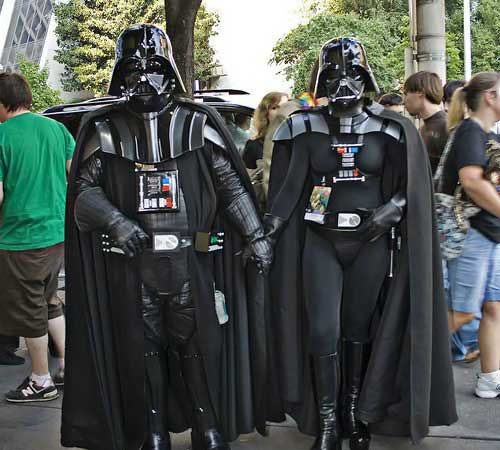## The Beauty (And Enigma) Of Math…

This Is Spooky…

1 x 8 + 1 = 9
12 x 8 + 2 = 98
123 x 8 + 3 = 987
1234 x 8 + 4 = 9876
12345 x 8 + 5 = 98765
123456 x 8 + 6 = 987654
1234567 x 8 + 7 = 9876543
12345678 x 8 + 8 = 98765432
123456789 x 8 + 9 = 987654321

1 x 9 + 2 = 11
12 x 9 + 3 = 111
123 x 9 + 4 = 1111
1234 x 9 + 5 = 11111
12345 x 9 + 6 = 111111
123456 x 9 + 7 = 1111111
1234567 x 9 + 8 = 11111111
12345678 x 9 + 9 = 111111111
123456789 x 9 +10= 1111111111

9 x 9 + 7 = 88
98 x 9 + 6 = 888
987 x 9 + 5 = 8888
9876 x 9 + 4 = 88888
98765 x 9 + 3 = 888888
987654 x 9 + 2 = 8888888
9876543 x 9 + 1 = 88888888
98765432 x 9 + 0 = 888888888

Brilliant, isn’t it?

And look at this symmetry:

1 x 1 = 1
11 x 11 = 121
111 x 111 = 12321
1111 x 1111 = 1234321
11111 x 11111 = 123454321
111111 x 111111 = 12345654321
1111111 x 1111111 = 1234567654321
11111111 x 11111111 = 123456787654321
111111111 x 111111111=12345678987654321

## Now, take a look at this…

What Equals 100%? What does it mean to give MORE than 100%? Ever wonder about those people who say they are giving more than 100%? We have all been to those meetings where someone wants you to give over 100%. How about achieving 103%? What equals 100% in life?

If:
A B C D E F G H I J K L M N O P Q R S T U V W X Y Z

is represented as:
1 2 3 4 5 6 7 8 9 10 11 12 13 14 15 16 17 18 19 20 21 22 23 24 25 26.

Then:

H-A-R-D-W-O-R- K
8+1+18+4+23+15+18+11 = 98%

and

K-N-O-W-L-E-D-G-E
11+14+15+23+12+5+4+7+5 = 96%

But,

A-T-T-I-T-U-D-E
1+20+20+9+20+21+4+5 = 100%

And,

B-U-L-L-S-H-I-T
2+21+12+12+19+8+9+20 = 103%

AND, look how far ass-kissing will take you.

A-S-S-K-I-S-S-I-N-G
1+19+19+11+9+19+19+9+14+7 = 118%

Therefore, one can conclude with mathematical certainty that:
While Hard work and Knowledge will get you close, and Attitude will get you there, it’s the Bullshit and Ass-kissing that will put you over the top !!!!!!

Don’t Believe It?… Break Out Your Calculator!

And Our Friend Sue From Tacoma Adds This Little Tidbit To An Already Interesting Piece…

L-O-V-E-O-F-G-O-D

12+15+22+5+15+6+7+15+4 = 101%

And the old FourEyes is now officially freaked out!!

Did you Stumble Here?? Check Out Our Other Goodies And Discover Stumble Something For Yourself!

## Darth And Dorothy… Matched At eHarmony.com On 10/31/06… Became Mr and Mrs Vader On 6/21/08…